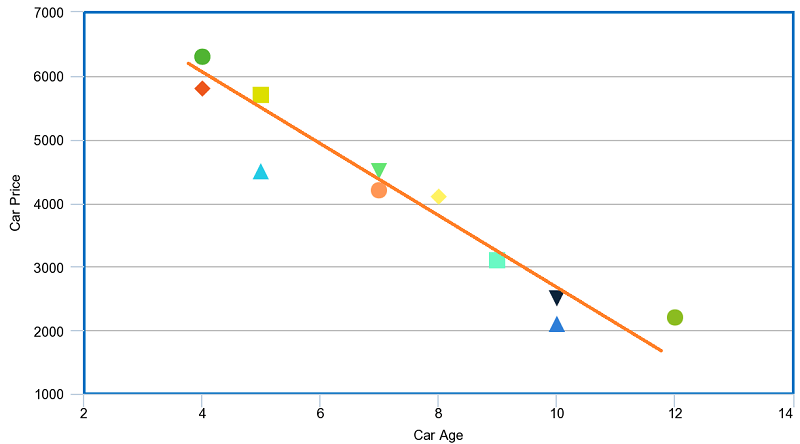# What Does a Scatter Plot Show?

If you are wondering what does a scatter plot show, the answer is more simple than you might think. The scatter plot has also other names such as scatter diagram, scatter graph, and correlation chart.

Scatter plot helps in many areas of today world – business, biology, social statistics, data science and etc.

On this page:

• What is scatter plot? Definition.
• What is the purpose of a scatter plot?
• When to use it?
• Types of correlation in a scatter plot.
• Advantages and disadvantages.

Let’s define it!

It is an X-Y diagram that shows a relationship between two variables. It is used to plot data points on a vertical and a horizontal axis. The purpose is to show how much one variable affects another.

Example:

A classic example is the relationship between monthly sales and advertising dollars in a company. The below table presents data for  7 online stores, their monthly e-commerce sales, and online advertising costs for the last year.

 Online Store Monthly E-commerce Sales (in 1000 s) Online Advertising Dollars (1000 s) 1 368 1.7 2 340 1.5 3 665 2.8 4 954 5 5 331 1.3 6 556 2.2 7 376 1.3

Now, let’s create the scatter diagram based on the data we have.The scatter plot shows that there is a relationship between monthly e-commerce sales (Y) and online advertising costs (X). More advertising costs lead to more sales.

The orange line you see in the plot is called “line of best fit” or a “trend line”. This line is used to help us make predictions that are based on past data.

Usually, when there is a relationship between 2 variables, the first one is called independent. The second variable is called dependent because its values depend on the first variable.

But it is also possible to have no relationship between 2 variables at all.

So, What is The Purpose of a Scatter Plot?

In today world of data science, Scatter graphs have a couple of purposes. Let’s list them:

• To show whether 2 variables are related or not.
• To show how much one variable affects another – the main purpose!
• To help you predict the behavior of one variable (dependent) based on the measure of the other variable (independent).

When To Use A Scatter Plot?

Scatter diagram has many applications and usages nowadays. Here are some of them:

• When trying to find out whether there is a relationship between 2 variables.
• When having paired numerical data.
• When working with root cause analysis tools to identify the potential for problems.
• When just want to visualize the correlation between 2 large datasets without regard to time.

Types of Correlation in a Scatter Plot

In the above text, we many times mentioned the relationship between 2 variables. Thi is called correlation.

Ther are 3 types of correlation:

1. Positive Correlation

When one variable (dependent variable) increase as the other variable (independent variable) increases, there is a positive correlation. Height and clothes size is a good example here. When the height of a child increase, the clothes size also increase.

Another common example is the correlation between height and weight.

Visually, the positive correlation looks like that:As you see in the positive correlation, the “best-fit line” goes from the origin out to high Y- and X- values.

2. Negative correlation

As you might guess, we have negative correlation when the increase of one variable leads to decrease in the other. Car age and car price are correlating negatively. Usually, when car age increase, the car price decrease.

Let’s see how the Scatter plot looks like:As you see in the negative correlation, the trend line goes from a high-value on the y-axis down to a high-value on the x-axis.

3. No correlation

No correlation means there is no relationship between the variables. For example, there is no correlation between a child’ clothes size and his/her grades at school.

A Scatter graph without correlation looks like that:The above graphs are made by www.meta-chart.com/

Scatter plots aren’t one of the most often used visualization type of charts, but they have an important role. They show you large quantities of data and present a correlation between variables.

In addition to that, they are a valuable tool for working with linear regression models.

As everything else in this world, Scatter plots have some pros and cons:

Advantages of Scatter plots:

• Show a relationship and a trend in the data relationship.
• Show all data points, including minimum and maximum and outliers.
• Can highlight correlations.
• Retains the exact data values and sample size.
• Shows both positive and negative type of graphical correlation.

Disadvantages of Scatter Plots:

• Flat best-fit line gives inconclusive results.
• Interpretation can be subjective.
• Correlation does not mean and not show causation.
• Data on both axes have to be continuous data (see our post discrete vs continuous data).
• You cannot use Scatter diagrams to show the relation of more than two variables.

It is true that Scatter plots have some limitations. However, when used correctly, they are a great tool for overviews and showing patterns and relationship between some datasets.

If you need some real-life examples of how Scatter charts work, check our post simple linear regression examples.